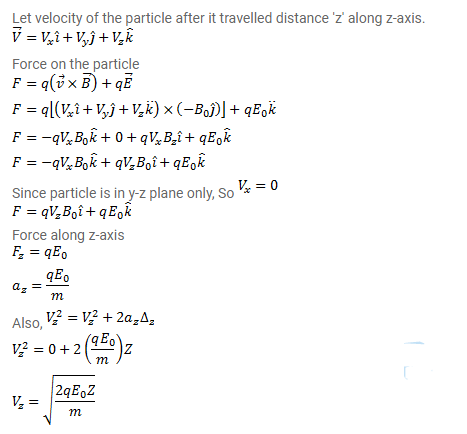# A particle having a mass m and charge q is released from

Question:

A particle having a mass $m$ and charge $q$ is released from the origin in a region in which electric field and magnetic field are given by $\vec{B}=-B_{0} \vec{j}$ and $\vec{E}=E_{0} \vec{k}$. Find the speed of the particle as a function of its z-coordinate.

Solution: